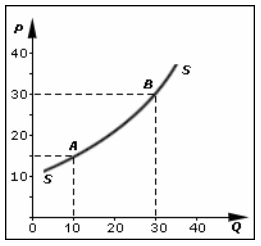# Supply Curve: Meaning, Shifting of Supply Curve, Movement Along Curve

The compilation of these The Theory of Firm Under Perfect Competition Notes makes students exam preparation simpler and organised.

## Supply Curve of a Firm

A diagrammatic scheme of things always makes the process of analyzing and observation easier. Justifiably, the supply curve graphically represents the producer’s whereabouts in the market. A supply curve is used to quickly draw conclusions about the supply of a commodity and the changes in it. So without further ado let us jump right into it.

### What is Supply?

Technically, supply refers to the quantity of a commodity that a firm is willing and able to supply at a given period of time, at a given price. Observe that this definition has four essential dimensions- quantity of a commodity, willingness to sell, price of the commodity, and period of time.

### Supply Curve

The supply curve is the locus of all the points showing various quantities of a commodity that a producer is willing to sell at various levels of prices, during a given period of time, assuming no change in other factors. Unlike a demand curve, the supply curve slopes upwards. This indicates the direct relationship between the quantity of a commodity supplied and its price, keeping other factors constant. The shape of the curve points out the characteristics of a firm.

Also, a supply curve is a good measure of comparison. One can simply look at the supply curves of two firms and distinguish between them. Alternatively, supply curves are also the graphical representation of supply schedules. A supply schedule is the tabular statement of the various quantities of a commodity that a firm is willing and able to sell at various levels of price during a given period of time. In essence, a supply curve can also be understood as the graphical counterpart of a supply schedule.

### Effects on Supply Curve

We are now aware of the various determinants of supply curves. An alteration of any of these factors has effects on the supply curve. We classified the determinants of supply into two categories- price and factors other than price.

Interestingly, the incidence of the alterations in determinants of supply is also categorized into two. The changes in price and factors other than price have varying consequences on the curve. These are classified as the movement along the supply curve and shift in the supply curve.

### Movement along the Supply Curve

When the price of commodity changes, other factors kept constant, the quantity supplied of commodity changes suitably. This is because of the direct relationship between the two. This is known as a change in quantity supplied. Graphically it causes movement along the supply curve. A change in price either causes supply curves to expand or contract.

If the prices increase, other factors kept constant, there is an increase in the quantity supplied which is referred to as an expansion in supply. Graphically, this is represented as an upward movement along the same supply curve.

Conversely, if the prices decrease, keeping other factors constant, firms tend to decrease the supply. This is referred to as a contraction in supply. Graphically, this is represented as a downward movement along the same supply curve.### Shifting of the Supply Curve

The factors other than price affect the supply curve in a different manner. These factors cause the supply curve to shift. Of course, this shift is also categorized into two which are- a leftward and rightward shift.

Note that, this shift occurs because the price is constant when studying the effect of other factors on supply. A rightward shift indicates a positive effect on the curve whereas a leftward shift indicates a negative effect on the supply curve. We have already studied the various factors other than price and their relationship with the supply of a commodity. The factors can either have a direct or an inverse relationship with the quantity of commodity supplied.Direct Relationship with Supply
Factors like the state of technology have a direct relationship with the supply. In other words, the change in such factors and supply occurs in the same direction. As a result, an increase in such factors leads to a shift in the rightward direction. Conversely, a decrease in such factors causes the curve to shift towards the left.

Inverse Relationship with Supply
Factors like the price of other goods tend to be in an inverse relationship with the supply of a commodity. Simply put, a change in such factors and supply occurs in opposite direction. A negative change in such factors induces a positive change in supply and vice versa. In effect, an increase in such factors leads to a shift in a leftward direction. However, a decrease of such factors causes the supply curve to shift towards the right.

Example:

Question:
Explain the effect of an increase in taxes on a supply curve.